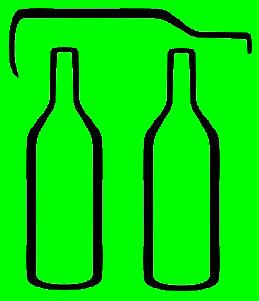# WinePi.comWhat is the real value of WinePi?

It's a rational number, that is, it is the result of the division of two integers.

We recognize 25.4 mm per inch (another definition).

And, we recognize 9 liters per case (12 bottles X 750 ml per bottle).

So, WinePi = 9 liters X 1,000 ml / liter X (1 cm3 / 1 ml) X (1 in / 2.54 cm)3 X 1 gallon / 231 in3

So, in the numerator, we have 9 X 1000

and in the denominator, we have 2.54 X 2.54 X 2.54 X 231,

or, let's restate

the numerator as 9 X 1,000 X 100 X 100 X 100

and the denominator as 254 X 254 X 254 X 231.

We can factor the numerator as 32 X 59X 29=9,000,000,000

and the denominator as 11 X 7 X 3 X 1273 X 23=3,785,411,784.

Or, we can simplify the numerator to 3 X 59X 26=375,000,000

and the denominator to 11 X 7 X 1273 = 157,725,491.

Using normal calculations gives a value of 2.377548471

Using double precision gives a value of 2.377548471223335996

Since this is rational, it must be repeating or it must be terminating.  It turns out WinePi is repeating, with a repeat sequence of 677,418 digits.  Here are the the first digits of WinePi.

Note that this can be calculated manually, or by careful programming.  Note that at no time does the remainder have more than 12 digits, so double precision will work.  For details, contact the author.

How many digits of WinePi should you use?  It depends...

This is the home site for Mike Von der Porten,

the WinePi Guy.

WinePi Home

###### last updated:  08/13/11 07:27:57 PM